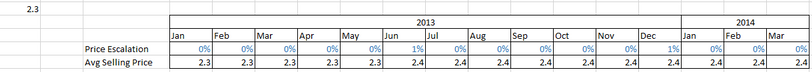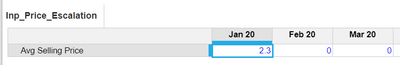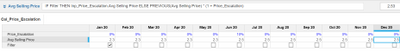# Computation with Price EscalationHi Guys,

I would like to ask something regarding a formula I have been working on. See the table below.Price Escalation formula = Input

Avg Selling Price = The Jan 13 value is constant referenced to 2.3 which is in another module. While the succeeding months starting Feb 13 to Mar 14 the formula is Avg Selling Price*(1+Price Escalation).

I tried the formula IF Price Escalation = 0 then Avg selling Price else Average Selling Price*Price Escalation.

I also tried IF Previous(Avg Selling Price)=0 then Avg Selling Price else Avg Selling Price*Price Escalation.

I still do not have the right calculation on my Line Item. Need a little input on this.

Appreciate it.

Regards,

Tagged:

•I am sure not about logic what you have explained. From the screenshot it looks like the formula is not correct for Avg selling price as well.

If i am assuming constant value to be 2.3 for Jan 13 and price escalation is 1% in Jun, how the average price will be 2.4 as per the formula you have provided.

1% of 2.3 =0.023 and in total it will be 2.323

Anyway i have tried to replicate :